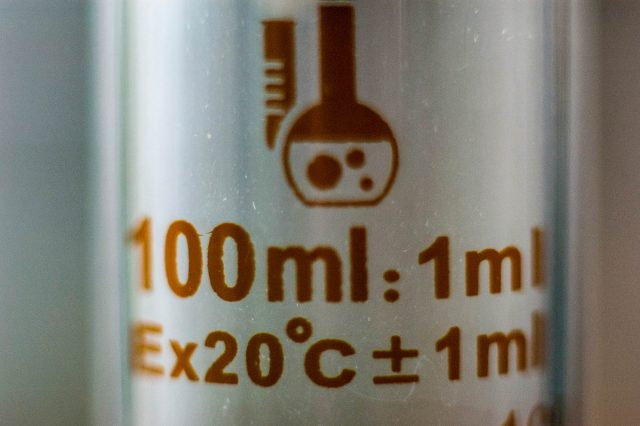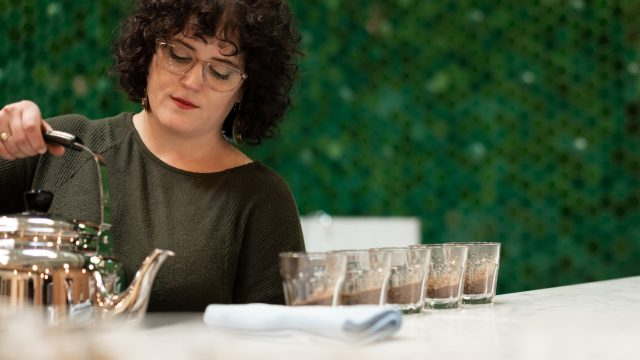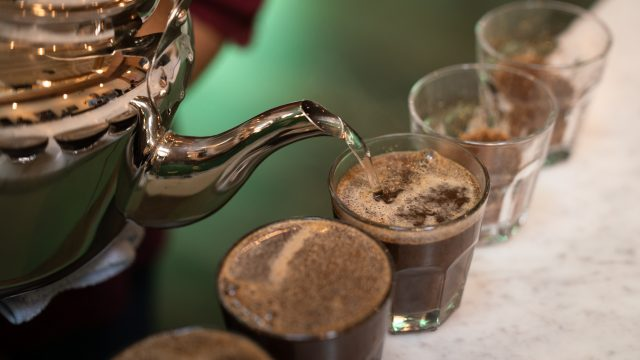Select Page

# Blending Water

### Some Important Math to Help You Mix Waters of Different TDS

Tasting Room Director Sandra Elisa Loofbourow and I recently ran into a difficult problem to solve at the Crown, and wanted to share some of the work it took to figure out how to mix two different waters to achieve a desired concentration and volume.

Our problem started with needing a specific water formula for an event.

We were using Third Wave Water which produces roughly 150ppm hardness when used with distilled water, and our tap water typically falls right around 60ppm. We wanted to avoid wasting money and plastic by purchasing distilled water, yet still have *roughly* calibrated H2O to the intended target TDS and get a total volume of 5 gallons.

Important Caveat: This will not address the actual mineral content. That topic is covered in detail in this two part series from 2018: Treading Water: A Practical H2O Guide for Coffee Professionals Part I Chemistry & Conditioning & Part II Sensory Data. We’re not going to speak to that today, we’re only concerned with the total mineral concentration.

With some help from Kathy Kornman, a physics and math teacher who is also my mother (Hi Mom!), we settled on a set of formulas to make a volume of water at a desired concentration.# First, Let’s Define Some Abbreviations:

• g = Goal Water
• h = High TDS water
• l = Low TDS water
• V = Volume. Let’s use gallons today.
• C = Concentration. We’re using parts per million (ppm) today, but you can use a percentage or another concentration unit

Now, Let’s Concentrate

There are two ways to approach this problem, and for both you’ll need to know in advance the concentrations of your high and low water, as well as your target TDS (which must be in between the two).

You’ll also want to know either a volume goal (Method 1) or a volume of at least one of the components (Method 2). There’s a quick additional formula in Method 2 that allows for you to scale up your proportions easily.

## Method 1 – Creating a Desired Volume & Concentration

If you know how much total water you need, you can create it using two similar equations.

Low TDS water volume can be found using this formula:
lV = gv * [(hC – gC ) ⁄ (hC – lC)]

High TDS water volume can be found using this formula:
hV = gv * [(gC – lC ) ⁄ (hC – lC)]

In our problem at the Crown, we knew we had to reach 150 TDS (our gC) at 5 gallons (gv). We had 60 TDS tap water (lC) and we knew we could make Third Wave Water with our tap water: 150 TDS + 60 TDS in 1 gallon increments = 210 TDS (hC).

Low TDS volume = 5 gallons * [(210 ppm -150 ppm)] / (210ppm – 60ppm)]
lV = 5 * (60/150)
lV = 2 gallons

High TDS volume = 5 gallons * [(150ppm – 60ppm) / (210ppm – 60ppm)]
hV = 5 * (90/150)
hV = 3 gallons

So, to get 5 gallons of water at 150 TDS we blend 2 gallons of 60 TDS water with 3 gallons of 210 TDS water. Or more simply, use 3 packets of Third Wave Water in 5 gallons of 60 TDS water.

## Method 2 – Creating a Desired Concentration Using a Known Component Volume

Approaching this problem from a different angle, we can still solve for an unknown component volume.

The thing about those Third Wave Water individual packets is that they are intended for 1 gallon of distilled water. If we’d blended 1 gallon (our hV) of our 60 TDS tap water with 1 Third Wave Water Packet in advance, then we’d have to dilute it using an unknown quantity of that same tap water. We need to solve for lV.

This formula is written in the form of an equation, where both sides are equal to each other. On the left side, we’ll add the product of our two waters’ volume and concentration. On the right, we’ll multiply the sum volumes of the two waters by our target TDS, our gC.

hV * hC + lV * lC = gC(hV + lV)

Essentially, what this says is that the volumes and concentrations of the two parts on the left, our components, will equal the final volume and concentration on the right, our target. This equation is actually the starting point for Method 1, but we’ll address that later. Right now, let’s plug in our numbers for Method 2.

Just to recap, we have 1 gallon (hV) of 210 ppm (hC) water. We need to make 150 ppm (gC) water using an unknown amount (lV) of 60 ppm water.

hV gallons * hC ppm + lV gallons * lC ppm = gC ppm (hV gallons + lV gallons)
1 gallon * 210ppm + lV gallons * 60ppm = 150ppm * (1gallon + lV gallons)

The only unknown is the volume of low concentration water. It’s our x. Let’s solve for it. I’m going to remove the units for now, but remember that we’re solving for volume in gallons.

Using the distributive property, we know that a*( b + c ) = (a * b) + ( a * c), so we can multiply that on the right side of the equation.

1 * 210 + lV * 60 = 150 * (1 + lV)
210 + 60x = 150 + 150lV

Now we just need to isolate lV.

210-150 = 150lV – 60lV
60 = 90lV
0.667 gallons = lV

So for every gallon of 200ppm water, we can add two thirds of a gallon of 60ppm to dilute it to 150ppm.

## Scaling Up Method 2 to a Desired Volume

For this particular situation, we needed 5 total gallons. To expand our fraction into the full volume, we need a little more math. We know our total yield with this formula is 1.667 gallons (the sum of the volumes of 1 gallon of high and 0.667 gallons of low water). Dividing our target volume 5 gallons by our yield of 1.667 gallons = 3 (essentially, how many 1.667 gallon units fit into 5 gallons).

So our units (u) can be found by dividing our goal volume by the sum of our high and low volumes.

gV / (hv + lV) = u
5 / (1 + 0.667) = 3

Now we take our units (3) and just multiply them by the high and low volumes.

u * hv + u * lV = gV
3 * 1 gallon of high + 3 * 0.667 gallons of low water = 5 gallons at 150ppm.
3 gallons + 2 gallons = 5 gallons at 150ppm.# Using Distilled Water?

Just for kicks, let’s try a different one to illustrate how having distilled water on hand can make this equation business a lot less complicated.

Let’s say we have super hard water, 666ppm (yikes!). We want to get back down to close to SCA specs, at 150, and we need 7 gallons total volume. How much distilled water (0 ppm) should we add?

### Method 1

Low TDS water volume:
lV = gv * [(hC – gC ) ⁄ (hC – lC)]
lV = 7 gallons * [(666 – 150)/(666-0)]
lV = 7 * (516/666)
lV = 5.423 gallons of distilled water

High TDS water volume:
hV = gv * [(gC – lC ) ⁄ (hC – lC)]
hV = 7 gallons * (150 – 0)/(666-0)
hV = 7 * (150)/(666)
hV = 1.576 gallons of water at 666 ppm

### Method 2

hV * hC + lV * lC = gC(hV + lV )
666ppm * 1 gallon + 0pmm * ?gallons = 150 ppm * (1gallon + ?gallons)

See where this is going? That 0ppm is doing some heavy lifting on the left side, eliminating one of our x factors. Let’s math this out real quick.

666 = 150 + 150lV
516 = 150lV
3.44 = lV

So, 3.44 gallons of distilled water added to 1 gallon of our high TDS tap will give us our desired concentration. Now how many units gets us to 7 gallons?

gV / (hv + lV) = u
7 / (1+3.44) = u
1.576 = u

So we’ll need 1.576 gallons at high TDS plus (3.44 * 1.567) = 5.423 gallons of distilled water to get 7 total gallons.# Why Does All This Work?

If you’re anything like me, you’ll want to know how this particular sausage is being made.

The key to unlocking these formulas is the substitution principle, which allows us to usefully replace an unknown variable with the function of a known one. Again, I’m grateful to Kathy Kornman here for breaking the steps into digestible portions for me.

The starting point is a simple equation based on what we know.

Chemistry tells us that the concentration of a solution is its solute’s mass divided by the liquid volume: C=m/V. Therefore a solute’s mass is equal to its volume multiplied by its concentration. m=C*V

We also know that the mass of our two components, when added together, equals the final mass.
hm + lm = gm

Now, measuring the mass of our solute (little ions of hard minerals like calcium and magnesium) is probably not the most efficient way to dilute a solution. So we substitute. If m=C*V, then we just drop in our concentration and volume figures and

hm + lm = gm
becomes
(hc * hv) + (lc * lv) = gc * gv

Concentration and volume — those are numbers we can work with. Concentration in parts per million and volume in gallons, or whatever units you prefer.

Method two is easy to get to from here, using substitution. We know that our component volumes, when added together, equal our target volume. hv + lv = gv

If we don’t need to worry about final volume, just concentration, we can drop this in as a substitute, and

(hc * hv) + (lc * lv) = gc * gv
becomes Method 2:
hV * hC + lV * lC = gC * (hV + lV)

It takes a little more creativity to get to Method 1, but the principle is the same. If we know everything except our two component volumes, we can still work them out individually because hv + lv = gv

Therefore
hv = gv – lv
And
lv = gv – hv

As a result, we can substitute one or the other to isolate a single variable and solve for it. Such that, if we’re looking for lv

(hc * hv) + (lc * lv) = gc * gv
Becomes
(hc * (gv – lv)) + (lc * lv) = gc * gv
Or if looking for hv it becomes
(hc * hv) + (lc * (gv – hv)) = gc * gv

When you work these two formulas down to isolate the low and high water volumes, that’s Method 1. I have the proof, as so many good ones are, scribbled on the back of napkin.

I won’t bore you with it. If you made it this far, you’ve probably already bitten off more than you bargained for! As Sandra asked me the other day, “Did you ever think you’d use so much math in your coffee career?”

No, I certainly did not.